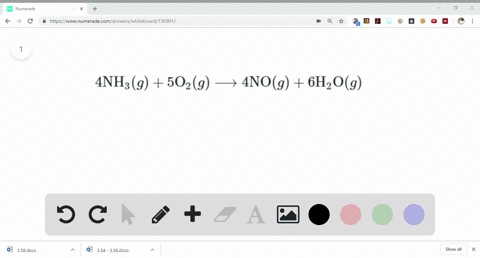Sign up for our free STEM online summer camps starting June 1st!View Summer Courses### One of the steps in the commercial process for co…

13:00University of New Mexico

Need more help? Fill out this quick form to get professional live tutoring.

Get live tutoring
Problem 79

The fizz produced when an Alka-Seltzer tablet is dissolved in water is due to the reaction between sodium bicarbonate $\left(\mathrm{NaHCO}_{3}\right)$ and citric acid $\left(\mathrm{H}_{3} \mathrm{C}_{6} \mathrm{H}_{5} \mathrm{O}_{7}\right) :$
\begin{aligned} 3 \mathrm{NaHCO}_{3}(a q)+\mathrm{H}_{3} \mathrm{C}_{6} \mathrm{H}_{5} \mathrm{O}_{7}(a q) & \longrightarrow \\ & 3 \mathrm{CO}_{2}(g)+3 \mathrm{H}_{2} \mathrm{O}(l)+\mathrm{Na}_{3} \mathrm{C}_{6} \mathrm{H}_{5} \mathrm{O}_{7}(a q) \end{aligned}
In a certain experiment 1.00 g of sodium bicarbonate and 1.00 g of citric acid are allowed to react. (a) Which is the limiting reactant? (b) How many grams of carbon dioxide form? (c) How many grams of the excess reactant remain after the limiting reactant is completely consumed?

a) See explanation for solution.
b) See work for solution.
c) Click for solution.

## Discussion

You must be signed in to discuss.

## Video Transcript

let us start. So for option A, we need to have a reaction between any Ex CEO three on C six. It's three Etch five or seven. Reaction can be presented as follows. Three on me, which seal three plus h three c six. It's five was seven gives us three carbon dioxide less three water, a long grid and they three C six. Etch five. Who was seven. Now? To calculate the molar mass, we need to have more the mask off. Both devi accidents as represented over here sodium for 23 hydrogen one carbon 12 on oxygen for three into 16. Same has to be done for the second reactor. Hi. Lo gin one carbon dioxide, six into 12. Oxygen one into five. Oxygen is one oxygen. Oxygen is one. Sorry. Hydrogen is wanting to five on oxygen is 7 to 16 further along. We need to deter mined the limiting the agent. So as it represented over here, three more off any Xia tree reacts with one moon off at three. C six h five or seven. Using the unitary method, we gonna have the limiting the agent as 0.762 grams off X tree. See six ej five or seven. But But we are so why did well one ground off limiting the agent that with x three c six match five or seven. So for this case and me Etch, See or three will act us limiting the agent. No for this holding the question we need to calculate the amount off Carbon dioxide form the moon. Thomas off carbon is 12 by oxygen is 16. The mullahs moss off full. Carbon dioxide is 44 grams here, three into 84.1 grams off limiting The agent reacts to produce three into 44 grams off carbon dioxide. So again, using the previous matter, assume the mass off carbon dioxide form will be 0.5 to 4 rounds on the end for option C. We need to calculate the mass off access reacted remaining after the completion off reaction. So the month off, its three C six ej five or seven remaining will be were provided with one gram minus minus 0.76 two rums, which gives us a little point 23 It groans off reactant remaining